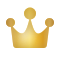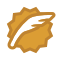# python tsfresh特征中文详解（更新中）

tsfresh是开源的提取时序数据特征的python包，能够提取出超过64种特征，堪称提取时序特征的瑞士军刀。最近有需求，所以一直在看，目前还没有中文文档， 有些特征含义还是很难懂的，我把我已经看懂的一部分放这，没看懂的我只写了标题，待我看懂我添加注解。

#### tsfresh.feature_extraction.feature_calculators.abs_energy(x)

$E = \sum_{i=1,...,n}x_i^2$

#### tsfresh.feature_extraction.feature_calculators.absolute_sum_of_changes(x)

$\sum_{i=1,...,n-1} | x_{i+1} - x_i|$

#### tsfresh.feature_extraction.feature_calculators.agg_autocorrelation(x, param)

$\frac{1}{n-1} \sum_{i=1,...,n} \frac{1}{(n-l)\sigma^2} \sum_{t=1}^{n-l}(X_t -\mu)(X_{t-1} -\mu)$
n是时间序列 $X_i$ 的长度，$\sigma^2$ 是方差，$\mu$表示均值

#### tsfresh.feature_extraction.feature_calculators.agg_linear_trend(x, param)

Parameters: x (pandas.Series) – the time series to calculate the feature of
param (list) – contains dictionaries {“attr”: x, “chunk_len”: l, “f_agg”: f} with x, f an string and l an int
Returns: the different feature values
Return type: pandas.Series

#### tsfresh.feature_extraction.feature_calculators.autocorrelation(x, lag)

$\frac{1}{(n-l)\sigma^2} \sum_{t=1}^{n-l}(X_t - \mu)(X_{t+l}-\mu)$

#### tsfresh.feature_extraction.feature_calculators.binned_entropy(x, max_bins)

$\sum_{k=0}^{min(max\_bins, len(x))} p_k log(p_k) \cdot\mathbf{1}_{(p_k > 0)}$
$p_k$ 表示落在第k个桶中的数占总体的比例。

max_bins (int) 桶的数量

#### tsfresh.feature_extraction.feature_calculators.c3(x, lag)

$\frac{1}{n-2lag} \sum_{i=0}^{n-2lag} x_{i + 2 \cdot lag}^2 \cdot x_{i + lag} \cdot x_{i}$

$\mathbb{E}[L^2(X)^2 \cdot L(X) \cdot X]$

#### tsfresh.feature_extraction.feature_calculators.change_quantiles(x, ql, qh, isabs, f_agg)

Parameters:
x (pandas.Series) – 时序数据
ql (float) – 分位数的下限
qh (float) – 分位数的上线
isabs (bool) – 使用使用绝对值
f_agg (str, name of a numpy function (e.g. mean, var, std, median)) – numpy自带的聚合函数（均值，方差，标准差，中位数）

#### tsfresh.feature_extraction.feature_calculators.cid_ce(x, normalize)

$\sqrt{ \sum_{i=0}^{n-2lag} ( x_{i} - x_{i+1})^2 }$

#### tsfresh.feature_extraction.feature_calculators.cwt_coefficients(x, param)

$\frac{2}{\sqrt{3a} \pi^{\frac{1}{4}}} (1 - \frac{x^2}{a^2}) exp(-\frac{x^2}{2a^2})$

#### tsfresh.feature_extraction.feature_calculators.energy_ratio_by_chunks(x, param)

Calculates the sum of squares of chunk i out of N chunks expressed as a ratio with the sum of squares over the whole series.

Takes as input parameters the number num_segments of segments to divide the series into and segment_focus which is the segment number (starting at zero) to return a feature on.

If the length of the time series is not a multiple of the number of segments, the remaining data points are distributed on the bins starting from the first. For example, if your time series consists of 8 entries, the first two bins will contain 3 and the last two values, e.g. [ 0., 1., 2.], [ 3., 4., 5.] and [ 6., 7.].

Note that the answer for num_segments = 1 is a trivial “1” but we handle this scenario in case somebody calls it. Sum of the ratios should be 1.0.

###### Parameters:

x (numpy.ndarray) – the time series to calculate the feature of
param – contains dictionaries {“num_segments”: N, “segment_focus”: i} with N, i both ints

###### Returns:

the feature values

###### Return type:

list of tuples (index, data)

#### tsfresh.feature_extraction.feature_calculators.fft_aggregated(x, param)

Returns the spectral centroid (mean), variance, skew, and kurtosis of the absolute fourier transform spectrum.

###### Parameters:

x (numpy.ndarray) – the time series to calculate the feature of
param (list) – contains dictionaries {“aggtype”: s} where s str and in [“centroid”, “variance”, “skew”, “kurtosis”]

###### Returns:

the different feature values

###### Return type:

pandas.Series

This function is of type: combiner

#### tsfresh.feature_extraction.feature_calculators.fft_coefficient(x, param)

Calculates the fourier coefficients of the one-dimensional discrete Fourier Transform for real input by fast fourier transformation algorithm

$A_k = \sum_{m=0}^{n-1} a_m \exp \left \{ -2 \pi i \frac{m k}{n} \right \}, \qquad k = 0,\ldots , n-1.$

The resulting coefficients will be complex, this feature calculator can return the real part (attr==”real”), the imaginary part (attr==”imag), the absolute value (attr=”“abs) and the angle in degrees (attr==”angle).

###### Parameters:

x (numpy.ndarray) – the time series to calculate the feature of
param (list) – contains dictionaries {“coeff”: x, “attr”: s} with x int and x >= 0, s str and in [“real”, “imag”, “abs”, “angle”]

###### Returns:

the different feature values

###### Return type:

pandas.Series

This function is of type: combiner

#### tsfresh.feature_extraction.feature_calculators.friedrich_coefficients(x, param)

Coefficients of polynomial h(x), which has been fitted to the deterministic dynamics of Langevin model
$\dot{x}(t) = h(x(t)) + \mathcal{N}(0,R)$

as described by .

For short time-series this method is highly dependent on the parameters.

References

 Friedrich et al. (2000): Physics Letters A 271, p. 217-222
Extracting model equations from experimental data

###### Parameters:

x (numpy.ndarray) – the time series to calculate the feature of
param (list) – contains dictionaries {“m”: x, “r”: y, “coeff”: z} with x being positive integer, the order of polynom to fit for estimating fixed points of dynamics, y positive float, the number of quantils to use for averaging and finally z, a positive integer corresponding to the returned coefficient

###### Returns:

the different feature values

pandas.Series

#### tsfresh.feature_extraction.feature_calculators.large_standard_deviation(x, r)

$std(x) > r * (max(X)-min(X))$

x的长度

#### tsfresh.feature_extraction.feature_calculators.linear_trend(x, param)

Calculate a linear least-squares regression for the values of the time series versus the sequence from 0 to length of the time series minus one. This feature assumes the signal to be uniformly sampled. It will not use the time stamps to fit the model. The parameters control which of the characteristics are returned.

Possible extracted attributes are “pvalue”, “rvalue”, “intercept”, “slope”, “stderr”, see the documentation of linregress for more information.

###### Parameters:

x (numpy.ndarray) – the time series to calculate the feature of
param (list) – contains dictionaries {“attr”: x} with x an string, the attribute name of the regression model

###### Returns:

the different feature values

###### Return type:

pandas.Series

This function is of type: combiner

#### tsfresh.feature_extraction.feature_calculators.max_langevin_fixed_point(x, r, m)

Largest fixed point of dynamics :math:argmax_x {h(x)=0}` estimated from polynomial h(x), which has been fitted to the deterministic dynamics of Langevin model
$\dot(x)(t) = h(x(t)) + R \mathcal(N)(0,1)$
as described by

Friedrich et al. (2000): Physics Letters A 271, p. 217-222 Extracting model equations from experimental data
For short time-series this method is highly dependent on the parameters.

###### Parameters:

x (numpy.ndarray) – the time series to calculate the feature of
m (int) – order of polynom to fit for estimating fixed points of dynamics
r (float) – number of quantils to use for averaging

###### Returns:

Largest fixed point of deterministic dynamics

float

#### tsfresh.feature_extraction.feature_calculators.mean_abs_change(x)

$\frac{1}{n} \sum_{i=1,\ldots, n-1} | x_{i+1} - x_{i}|$

#### tsfresh.feature_extraction.feature_calculators.mean_change(x)

$\frac{1}{n} \sum_{i=1,\ldots, n-1} x_{i+1} - x_{i}$

#### tsfresh.feature_extraction.feature_calculators.mean_second_derivative_central(x)

$\frac{1}{n} \sum_{i=1,\ldots, n-1} \frac{1}{2} (x_{i+2} - 2 \cdot x_{i+1} + x_i)$

#### tsfresh.feature_extraction.feature_calculators.number_crossing_m(x, m)

Calculates the number of crossings of x on m. A crossing is defined as two sequential values where the first value is lower than m and the next is greater, or vice-versa. If you set m to zero, you will get the number of zero crossings.

###### Parameters:

x (numpy.ndarray) – the time series to calculate the feature of
m (float) – the threshold for the crossing

###### Returns:

the value of this feature

int

#### tsfresh.feature_extraction.feature_calculators.number_cwt_peaks(x, n)

This feature calculator searches for different peaks in x. To do so, x is smoothed by a ricker wavelet and for widths ranging from 1 to n. This feature calculator returns the number of peaks that occur at enough width scales and with sufficiently high Signal-to-Noise-Ratio (SNR)

###### Parameters:

x (numpy.ndarray) – the time series to calculate the feature of
n (int) – maximum width to consider

###### Returns:

the value of this feature

int

#### tsfresh.feature_extraction.feature_calculators.partial_autocorrelation(x, param)

$\alpha_k = \frac{ Cov(x_t, x_{t-k} | x_{t-1}, \ldots, x_{t-k+1})} {\sqrt{ Var(x_t | x_{t-1}, \ldots, x_{t-k+1}) Var(x_{t-k} | x_{t-1}, \ldots, x_{t-k+1} )}}$

#### tsfresh.feature_extraction.feature_calculators.percentage_of_reoccurring_datapoints_to_all_datapoints(x)

len(different values occurring more than once) / len(different values)

#### tsfresh.feature_extraction.feature_calculators.range_count(x, min, max)

x中在min和max之间的数的个数

#### tsfresh.feature_extraction.feature_calculators.symmetry_looking(x, param)

$| mean(X)-median(X)| < r * (max(X)-min(X))$

#### tsfresh.feature_extraction.feature_calculators.time_reversal_asymmetry_statistic(x, lag)

$\frac{1}{n-2lag} \sum_{i=0}^{n-2lag} x_{i + 2 \cdot lag}^2 \cdot x_{i + lag} - x_{i + lag} \cdot x_{i}^2$

$\mathbb{E}[L^2(X)^2 \cdot L(X) - L(X) \cdot X^2]$

x中值等于value的计数

#### tsfresh.feature_extraction.feature_calculators.variance_larger_than_standard_deviation(x)

xindooCSDN认证博客专家 Linux 分布式 Spring

03-222750

#### sklearn.feature_extraction常用API介绍

05-271128

#### 基于tsfresh特征扩展和lasso时序建模09-071万+

#### 【时间序列 - 04】tsfresh：一种“提取时间序列特征”的包

01-10611

#### 自动提取时序特征 tsfresh(2)

06-131万+

#### 分析时序数据的三步骤：使数据平稳、时序模型、评估

02-248280

#### 机器学习教程 之 慢特征分析：时序特征挖掘

07-2821万+

#### SecureCRT的下载、安装（ 过程非常详细！！值得查看）

07-103万+

#### 如何理解自相关和偏自相关图(最全面的讲解)

03-052808

#### tsfresh 安装问题收集

10-293万+

#### 【安全】Web渗透测试（全流程）©️2020 CSDN 皮肤主题: 技术工厂 设计师: CSDN官方博客点击重新获取扫码支付1.余额是钱包充值的虚拟货币，按照1:1的比例进行支付金额的抵扣。
2.余额无法直接购买下载，可以购买VIP、C币套餐、付费专栏及课程。余额充值